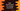# Dart program to find factorial of a number recursively# Dart program to find out the factorial of a number recursively :

The factorial is the product of a number and all the numbers less than it. For example, factorial of 4 is 4 * 3 * 2 * 1 i.e. 24. If you want to find the factorial programmatically, you can either use one loop and multiply all numbers starting from 2 to that number or you can use one recursive method.

In this tutorial, I will show you how to find the factorial of a number recursively.

## Recursive function :

A function is called recursive, if it calls itself. For example :

``````function_name(){
function_name();
}``````

Here, functionname_ function calls itself inside the body. Note that the recursive function should stop. You should add one condition that stops the function call at some point in time. Otherwise, it will run for an indefinite amount of time and your program will freeze.

### Find the factorial of a number recursively :

We can find the factorial of a number recursively. The dart program looks like as below :

``````import 'dart:io';

findFactorial(int no) {
if (no == 1) {
return 1;
}
return no * findFactorial(no - 1);
}

main() {
print("Enter a number : ");
``````Enter a number :